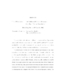## Exit Problem and Stochastic Resonance for a Class of Random Perturbations##### Files
umi-umd-2346.pdf(420.72 KB)
The asymptotic exit problems for diffusion processes with small parameter were considered in the classic work of Freidlin and Wentzell. In 2000, a mathematical theory of stochastic resonance for systems with random perturbations was established by Freidlin in the frame of the large deviation theory. This dissertation concerns exit problems and stochastic resonance for a class of random perturbations approximating white noise. The tools used in the proofs are the large deviation theory and the Markov property of the processes. The first problem considered is the exit problem and stochastic resonance for random perturbations of random walks. It turns out that a specific random walk can be chosen which approximates the large deviation asymptotics of the Wiener process in the best way. Analogous results concerning exit problems and stochastic resonance for this type of random perturbations were obtained under appropriate assumptions and compared with those of white noise type perturbation. The second problem I consider is the exit problems for random perturbations of a Gaussian process $\eta_{t}^{\mu,\varepsilon}$ which satisfies the equation $\mu \dot{\eta}_{t}^{\mu,\varepsilon}=- \eta_{t}^{\mu,\varepsilon}+\sqrt{\varepsilon}\dot{W}_{t}, \,\eta_{0}^{\mu,\varepsilon}=y, \,0&lt;\mu&lt;&lt;1,\,0&lt;\varepsilon&lt;&lt;1$. One can check that $\int_{0}^{t} \eta_{s}^{\mu,\varepsilon}ds$ converges to $\sqrt{\varepsilon}W_{t}$ uniformly on $[0,T]$ in probability as $\mu \downarrow 0$. Results concerning asymptotic exit problems for this type of random perturbation were obtained under appropriate assumptions. Since $\eta_{t}^{\mu,\varepsilon}$ is not a Markov process, this creates some difficulties for the proof. A new Markov process was constructed and the Markov property of the new process was used in the proof.